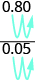## Writing Ratios as Fractions

### Learning Outcomes

• Given a ratio of two integers, write it as a fraction
• Given a ratio of two decimals, write it as a fraction
• Given a ratio of two mixed numbers, write it as a fraction

When you apply for a mortgage, the loan officer will compare your total debt to your total income to decide if you qualify for the loan. This comparison is called the debt-to-income ratio. A ratio compares two quantities that are measured with the same unit. If we compare $a$ and $b$ , the ratio is written as $a\text{ to }b,\frac{a}{b},\text{or}\mathit{\text{a}}\text{:}\mathit{\text{b}}\text{.}$

### Ratios

A ratio compares two numbers or two quantities that are measured with the same unit. The ratio of $a$ to $b$ is written $a\text{ to }b,\frac{a}{b},\text{or}\mathit{\text{a}}\text{:}\mathit{\text{b}}\text{.}$

In this section, we will use the fraction notation. When a ratio is written in fraction form, the fraction should be simplified. If it is an improper fraction, we do not change it to a mixed number. Because a ratio compares two quantities, we would leave a ratio as $\frac{4}{1}$ instead of simplifying it to $4$ so that we can see the two parts of the ratio.

### example

Write each ratio as a fraction: ⓐ $15\text{ to }27$ ⓑ $45\text{ to }18$.

Solution

 ⓐ $\text{15 to 27}$ Write as a fraction with the first number in the numerator and the second in the denominator. $\frac{15}{27}$ Simplify the fraction. $\frac{5}{9}$
 ⓑ $\text{45 to 18}$ Write as a fraction with the first number in the numerator and the second in the denominator. $\frac{45}{18}$ Simplify. $\frac{5}{2}$

We leave the ratio in ⓑ as an improper fraction.

### try it

In the following video you will see more examples of how to express a ratio as a fraction.

## Ratios Involving Decimals

We will often work with ratios of decimals, especially when we have ratios involving money. In these cases, we can eliminate the decimals by using the Equivalent Fractions Property to convert the ratio to a fraction with whole numbers in the numerator and denominator.
For example, consider the ratio $0.8\text{ to }0.05$. We can write it as a fraction with decimals and then multiply the numerator and denominator by $100$ to eliminate the decimals.Do you see a shortcut to find the equivalent fraction? Notice that $0.8=\frac{8}{10}$ and $0.05=\frac{5}{100}$. The least common denominator of $\frac{8}{10}$ and $\frac{5}{100}$ is $100$. By multiplying the numerator and denominator of $\frac{0.8}{0.05}$ by $100$, we ‘moved’ the decimal two places to the right to get the equivalent fraction with no decimals. Now that we understand the math behind the process, we can find the fraction with no decimals like this:“Move” the decimal 2 places. $\frac{80}{5}$ Simplify. $\frac{16}{1}$

You do not have to write out every step when you multiply the numerator and denominator by powers of ten. As long as you move both decimal places the same number of places, the ratio will remain the same.

### example

Write each ratio as a fraction of whole numbers:
ⓐ $4.8\text{ to }11.2$
ⓑ $2.7\text{ to }0.54$

### try it

The following video shows more examples of how to express a ratio given as a decimal as a fraction.

Some ratios compare two mixed numbers. Remember that to divide mixed numbers, you first rewrite them as improper fractions.

### example

Write the ratio of $1\frac{1}{4}\text{ to }2\frac{3}{8}$ as a fraction.